## Wednesday, August 30, 2017

### Visualizing the effect of individual species values (& errors therein) on PCMs

At the recent Evolution 2017 meeting in Portland, Oregon, Revell Lab postdoc Klaus Schliep used a visualization that I thought was interesting. Basically, he visualized the effect of individual leaves (tips) on the estimated transition rate q01 for a continuous- time Markov model of discrete trait evolution on the tree (the Mk model).

It occurred to me that this could be applied to anything, such as, for instance, the calculation of phylogenetic signal, or the σ2 rate parameter from a Brownian model. This could help us identify errors in our tree or data, or simply understand how sensitive our inference is to any individual observation in our dataset.

This is what I mean.

First, for phylogenetic signal using Blomberg et al.'s K statistic.

``````library(phytools)
tree
``````
``````##
## Phylogenetic tree with 26 tips and 25 internal nodes.
##
## Tip labels:
##  A, B, C, D, E, F, ...
##
## Rooted; includes branch lengths.
``````
``````x
``````
``````##           A           B           C           D           E           F
## -0.30072600  0.36692028  0.02339083  0.28425390  0.08200686 -0.04357881
##           G           H           I           J           K           L
## -0.65405735 -0.34157952 -0.58171643 -0.25767099  0.45571102 -0.69751648
##           M           N           O           P           Q           R
## -1.29203896 -1.64489731 -0.86747689 -0.44377476 -0.52001290 -0.32956650
##           S           T           U           V           W           X
## -0.20614204 -0.56731102 -1.62216360 -0.79246466 -0.95126907 -0.98715081
##           Y           Z
## -1.08186062 -0.91121102
``````
``````obsK<-phylosig(tree,x)
obsK
``````
``````##  0.4922464
``````
``````foo<-function(tip,t,x,method="K"){
t<-drop.tip(t,tip)
x<-x[t\$tip.label]
obj<-phylosig(t,x,method)
if(is.list(obj)) obj<-obj\$lambda
obj
}
tips<-tree\$tip.label
K<-sapply(tips,foo,t=tree,x=x)
plotTree.barplot(tree,K,args.barplot=list(xlim=c(0,max(c(1,max(K)))),
xlab="K"))
abline(v=obsK,lty="dotted",col="red",lwd=2)
text(x=obsK,y=0.98*par()\$usr,"observed value of K",pos=4)
``````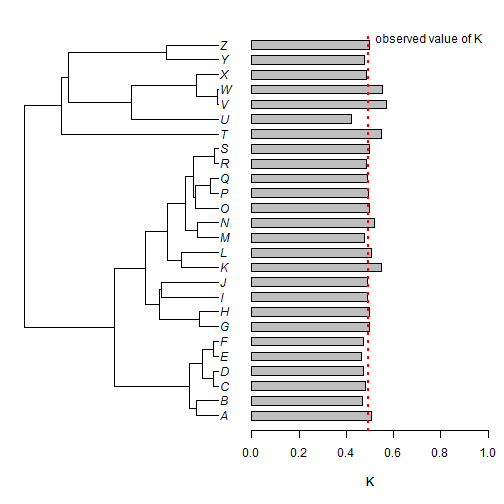Next, let's imagine that one of our observations, that of taxon `"W"` is off by 1 unit. Let's see what happens to our result:

``````x.err<-x
x.err["W"]<-x["W"]+1
K<-sapply(tips,foo,t=tree,x=x.err)
plotTree.barplot(tree,K,args.barplot=list(xlim=c(0,max(c(1,max(K)))),
xlab="K"))
abline(v=obsK,lty="dotted",col="red",lwd=2)
text(x=obsK,y=0.98*par()\$usr,"original value of K",pos=4)
abline(v=phylosig(tree,x.err),lty="dotted",col="blue",lwd=2)
text(x=phylosig(tree,x.err),y=0.5*par()\$usr,"observed value of K (with error)",pos=4)
``````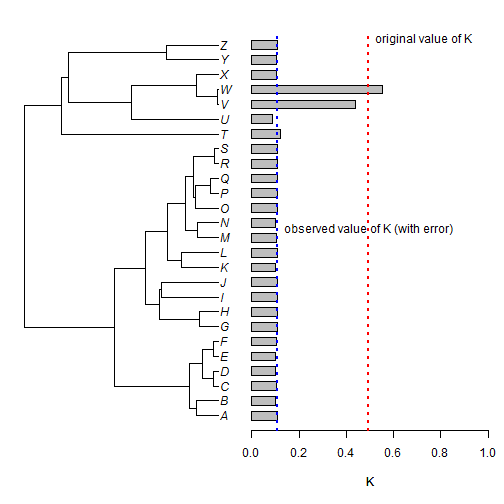You might have guessed that I didn't pick taxon `"W"` at random - it also happens to be at the end of a very short terminal edge. Let's see what happens if we do the same thing to a species that is less closely related to the others in the tree - say taxon `"T"`.

``````x.err<-x
x.err["T"]<-x["T"]+1
K<-sapply(tips,foo,t=tree,x=x.err)
plotTree.barplot(tree,K,args.barplot=list(xlim=c(0,max(c(1,max(K)))),
xlab="K"))
abline(v=obsK,lty="dotted",col="red",lwd=2)
text(x=obsK,y=0.98*par()\$usr,"original value of K",pos=4)
abline(v=phylosig(tree,x.err),lty="dotted",col="blue",lwd=2)
text(x=phylosig(tree,x.err),y=0.5*par()\$usr,
"observed value of K\n   (with error)",pos=4)
``````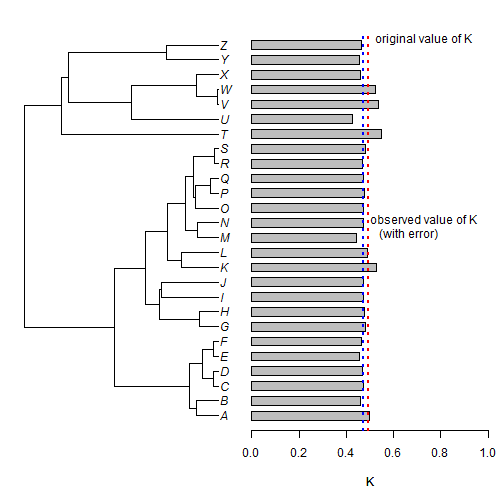Here, we can see that the same amount of error in our phenotypic value for taxon `"T"` has much less influence on the estimation of phylogenetic signal in our data.

OK, let's do the same thing now - but with the estimation of σ2 from a Brownian motion model. Here I'll use the function `evol.vcv`, because it is quite fast in this case - but we could also use `fitContinuous` from the geiger package.

``````obsSig2<-evol.vcv(tree,as.matrix(x))\$R[1,1]
obsSig2
``````
``````##  0.8490552
``````
``````foo<-function(tip,t,x){
t<-drop.tip(t,tip)
x<-x[t\$tip.label]
evol.vcv(t,as.matrix(x))\$R[1,1]
}
tips<-tree\$tip.label
sig2<-sapply(tips,foo,t=tree,x=x)
plotTree.barplot(tree,sig2,args.barplot=list(xlim=c(0,max(c(2,max(sig2)))),
xlab=expression(sigma^2)))
abline(v=obsSig2,lty="dotted",col="red",lwd=2)
text(x=obsSig2,y=0.98*par()\$usr,
expression(paste("observed value of ",sigma^2)),pos=4)
``````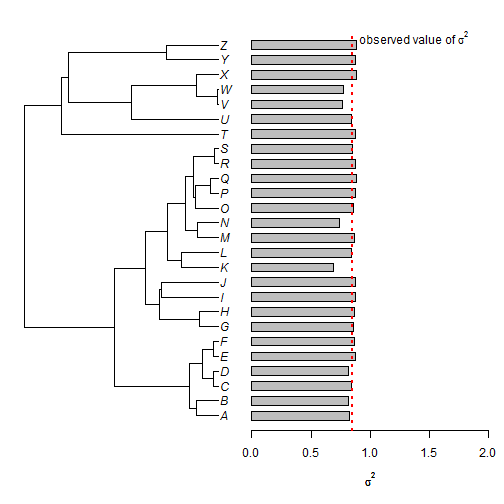Now with error:

``````x.err<-x
x.err["W"]<-x["W"]+1
sig2<-sapply(tips,foo,t=tree,x=x.err)
plotTree.barplot(tree,sig2,args.barplot=list(xlim=c(0,max(c(9,max(sig2)))),
xlab=expression(sigma^2),
main="effect of error in taxon W\n(when each taxon is excluded)",
cex.main=1))
abline(v=obsSig2,lty="dotted",col="red",lwd=2)
abline(v=evol.vcv(tree,as.matrix(x.err))\$R[1,1],lty="dotted",col="blue",lwd=2)
legend(x=4.5,y=0.98*par()\$usr,c(expression(paste("original ",sigma^2)),
expression(paste("observed ",sigma^2))),lty=rep("dotted",2),
lwd=rep(2,2),col=c("red","blue"),bty="n")
``````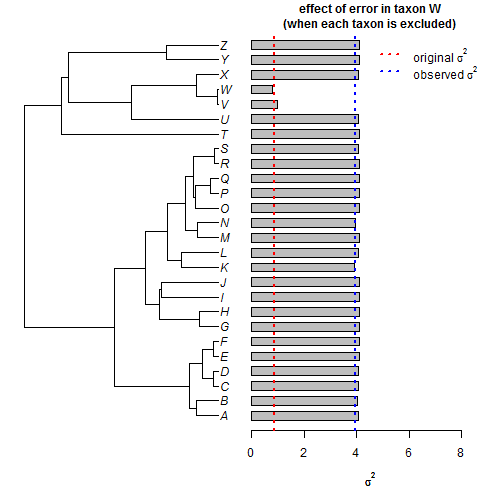``````x.err<-x
x.err["T"]<-x["T"]+1
sig2<-sapply(tips,foo,t=tree,x=x.err)
plotTree.barplot(tree,sig2,args.barplot=list(xlim=c(0,max(c(2,max(sig2)))),
xlab=expression(sigma^2),
main="effect of error in taxon T\n(when each taxon is excluded)",
cex.main=1))
abline(v=obsSig2,lty="dotted",col="red",lwd=2)
abline(v=evol.vcv(tree,as.matrix(x.err))\$R[1,1],lty="dotted",col="blue",lwd=2)
legend(x=1,y=0.98*par()\$usr,c(expression(paste("original ",sigma^2)),
expression(paste("observed ",sigma^2))),lty=rep("dotted",2),
lwd=rep(2,2),col=c("red","blue"),bty="n")
``````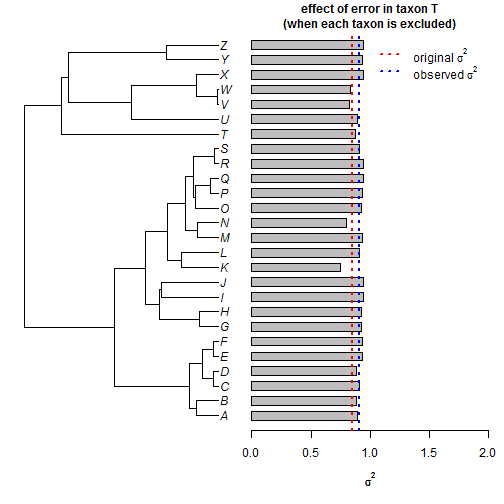As before, the weight of taxon `"W"`, and it's sister taxon `"V"`, is disproportionately high - in this case to increase the estimated value of σ2

I think there is more we could do with this type of visualization - including by dropping pairs of tips, for instance - but this is where I'll leave it for this evening.

The data for this exercise were simulated as follows:

``````tree<-pbtree(n=26,tip.label=LETTERS,scale=1)
x<-fastBM(tree)
``````